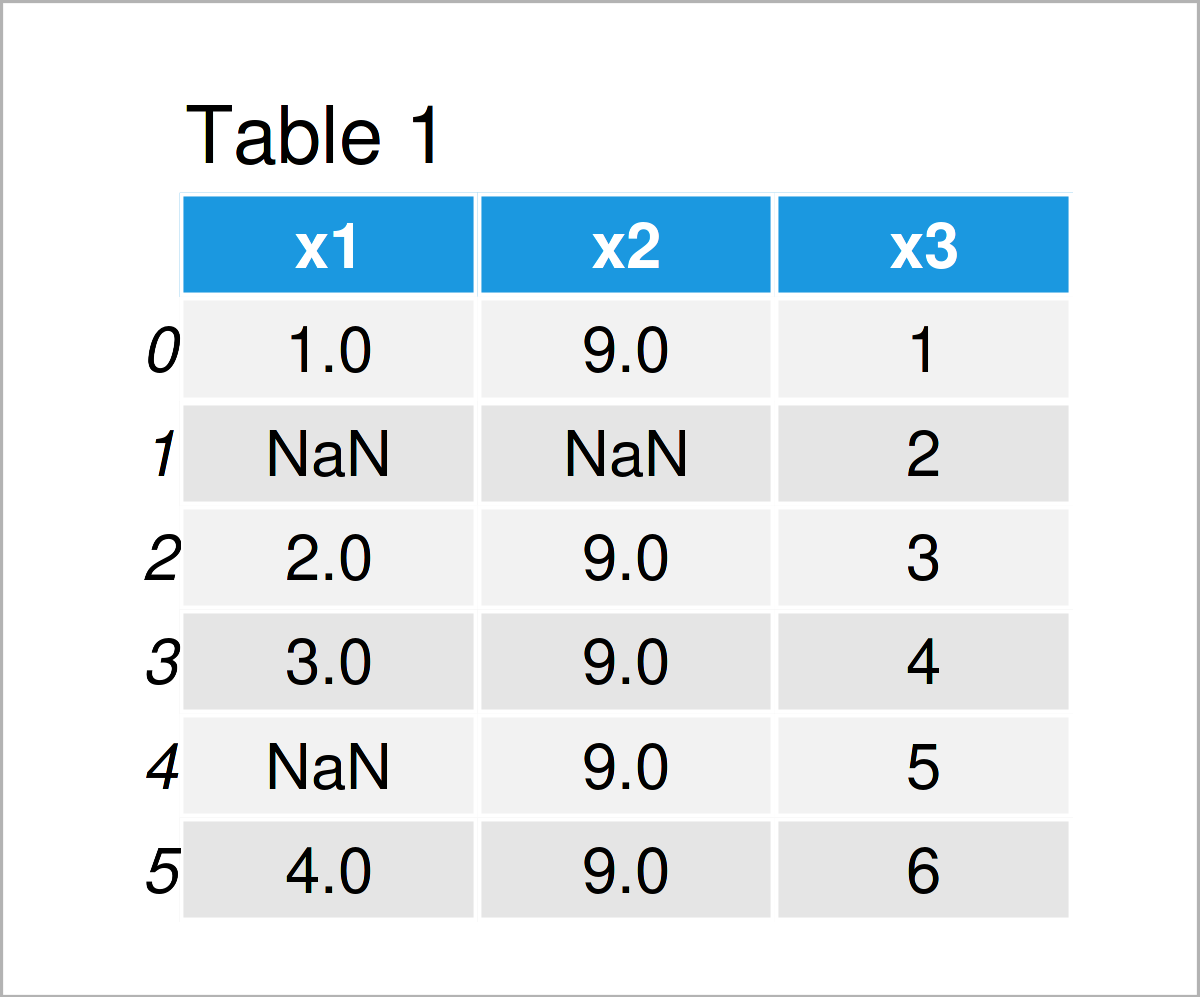# Count NaN Values in pandas DataFrame in Python (5 Examples)

In this tutorial you’ll learn how to get the number of NaN values in a pandas DataFrame in Python programming.

The post looks as follows:

Let’s get started…

## Example Data & Libraries

We first have to load the pandas library, in order to use the functions that are included in the library:

`import pandas as pd # Import pandas library to Python`

We’ll use the following data as basement for this Python tutorial:

```data = pd.DataFrame({'x1':[1, float('NaN'), 2, 3, float('NaN'), 4], # Create DataFrame 'x2':[9, float('NaN'), 9, 9, 9, 9], 'x3':range(1, 7)}) print(data) # Print example DataFrame```Table 1 shows the structure of our example data: It is made of six rows and three columns called “x1”, “x2”, and “x3”. Some of the variables in our data set contain NaN values (i.e. Not a Number).

## Example 1: Count NaN Values in All Columns of pandas DataFrame

In Example 1, I’ll explain how to return the number of NaN values in each column of a pandas DataFrame in Python.

For this, we can use the is.na and the sum functions as shown below:

```print(data.isna().sum()) # Number of NaNs by column # x1 2 # x2 1 # x3 0 # dtype: int64```

Have a look at the previous console output: It shows that our first column x1 contains two NaN values, the second column x2 contains one NaN value, and the third column x3 contains no NaN values.

## Example 2: Count NaN Values in One Specific Column of pandas DataFrame

Example 2 explains how to count the NaN values in a single DataFrame column.

The following Python code returns only the amount of NaNs in the column x1:

```print(data['x1'].isna().sum()) # Number of NaNs in one column # 2```

As you can see based on the previous output, the column x1 consists of two NaN values.

## Example 3: Count NaN Values in All Rows of pandas DataFrame

The following code shows how to count NaN values row wise.

To do this, we have to specify the axis argument within the sum function to be equal to 1:

```print(data.isnull().sum(axis = 1)) # Number of NaNs by row # 0 0 # 1 2 # 2 0 # 3 0 # 4 1 # 5 0 # dtype: int64```

The row with the index position 1 contains two NaN values and the row with the index position 4 contains one NaN value. The other rows do not contain any NaN values.

## Example 4: Count NaN Values in One Specific Row of pandas DataFrame

This example illustrates how to extract the number of NaN values only for one row by subsetting this row by its index position:

```print(data.loc[].isna().sum().sum()) # Number of NaNs in one row # 1```

The row with the index position 4 contains one NaN value.

## Example 5: Count All NaN Values in Entire pandas DataFrame

In this example, I’ll explain how to count all NaN values in the whole pandas DataFrame.

For this, we have to apply the sum function twice:

```print(data.isna().sum().sum()) # Number of NaNs in entire DataFrame # 3```

Overall, three cells of our pandas DataFrame are NaN.

## Video, Further Resources & Summary

Have a look at the following video on the YouTube channel of Minsuk Heo. He demonstrates how to check NaN and replace it in the video instruction.

Please accept YouTube cookies to play this video. By accepting you will be accessing content from YouTube, a service provided by an external third party.If you accept this notice, your choice will be saved and the page will refresh.

Furthermore, you might read the related tutorials on my website.

At this point you should have learned how to count the number of NaN values in a pandas DataFrame in Python. In case you have any further questions, don’t hesitate to let me know in the comments.

Subscribe to the Statistics Globe Newsletter